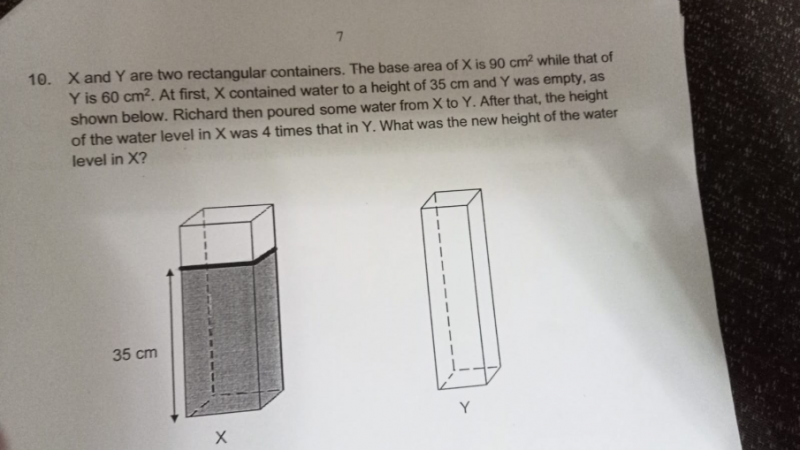# QuestionThank you.

X —>  Vol of water = 90 x 35 = 3150

Let h be the water level in container Y, then the new level of height in container X = 4h

Vol of water in Y = 60h

Vol of water in X = 90(4h) = 360h

360h + 60h = 3150

420h – 3150

h = 7.5

Hence the new height is 4 x 7.5 = 30cm

0 Replies 2 Likes ✔Accepted Answer